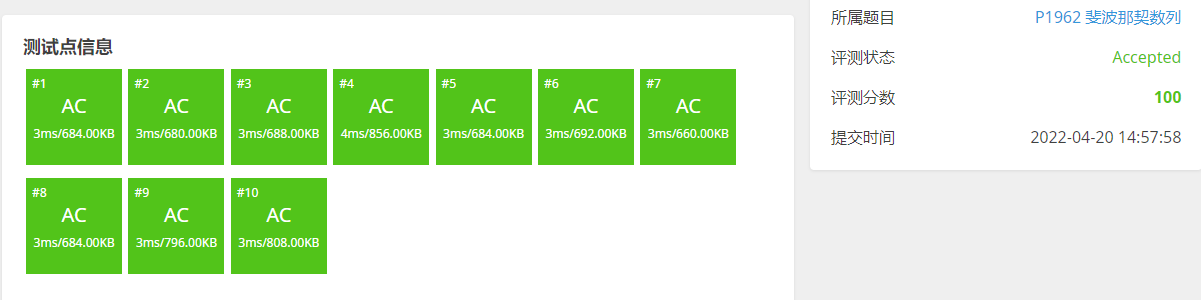## 题目描述

F_n = \left\{\begin{aligned} 1 \space (n \le 2) \\ F_{n-1}+F_{n-2} \space (n\ge 3) \end{aligned}\right.

## 输入输出样例

5


5


10


55


【数据范围】

## 题目分析

$\begin{bmatrix} f_{n-2} & f_{n-1} \end{bmatrix} \times \begin{bmatrix} 0 & 1\\ 1 & 1 \end{bmatrix} = \begin{bmatrix} f_{n-1} & f_{n-2}+f_{n-1} \end{bmatrix}= \begin{bmatrix} f_{n-1} & f_n \end{bmatrix}$

$\begin{bmatrix} 0 & 1\\ 1 & 1 \end{bmatrix}$

$A=\begin{bmatrix} 0 & 1\\ 1 & 1 \end{bmatrix}$

## 代码实现

#include <iostream>
#include <cstdio>
using namespace std;
typedef long long ll;
const int N=5;
const int M=1e9+7;
struct node{
ll a[N][N]={0};
int row,col;
};
node I;//单位矩阵

node matrixMins(node a,node b){//矩阵乘法
node c;//答案矩阵
c.row=a.row;
c.col=b.col;
int n=c.row,p=c.col,m=a.col;
//计算矩阵乘法
for(int i=1;i<=n;i++){
for(int j=1;j<=p;j++){
for(int k=1;k<=m;k++){
c.a[i][j]+=a.a[i][k]*b.a[k][j]%M;
c.a[i][j]%=M;
}
}
}
return c;
}
node matrixPow(node a,ll k){//矩阵的幂次方
if(k==0){// 0次方
return I;//矩阵的0次方是单位矩阵
}
node t=matrixPow(a,k/2);//求 a^{n/2} 次方
if(k&1){//判断k是否是奇数
return matrixMins(matrixMins(t,t),a);
}else{//k是偶数
return matrixMins(t,t);
}
}
int main(){
node a;
ll n;
cin>>n;
//处理斐波那契数列 递推矩阵
a.col=a.row=2;
a.a=0;
a.a=a.a=a.a=1;
//处理 单位矩阵
I.col=I.row=2;
I.a=I.a=1;
I.a=I.a=0;
//处理斐波那契数列初始值 [0 1]
node tt;
tt.row=1;tt.col=2;
tt.a=0;
tt.a=1;

node tmp=matrixPow(a,n);//计算A^n
node ans=matrixMins(tt,tmp);
cout<<ans.a;
return 0;
}Q.E.D.

••( ﾉ^ω^)ﾉﾟ 稻 花 香 里 说 丰 年 ， 听 取 WA 声 一 片 。(╥╯^╰╥)